Scientific Papers

# Predictive modeling of Alfvén eigenmode stability in inductive scenarios in JT-60SA## 1 Introduction

JT-60SA, a fully superconducting tokamak device jointly designed and constructed by Japan and Europe, is poised to support the experimental program of ITER as a satellite machine while paving the way for the future DEMO fusion reactor . By leveraging the legacies of JET and currently operating superconducting tokamaks such as WEST, EAST, and KSTAR, JT-60SA aims to extend the knowledge and capabilities of superconducting tokamaks. The primary missions of JT-60SA encompass two aspects. First, it aims to assist the ITER experimental program while providing complementary data and insights into key physics and engineering issues for DEMO reactors. Second, it aims to contribute to the development of DEMO by offering key information for the design of steady-state, advanced performance scenarios [4, 5]. Specifically, JT-60SA aims to achieve and control high-β, high-bootstrap current fraction (fBS) and high normalized plasma density (to Greenwald density) plasmas, a critical step toward economically viable steady-state DEMO reactors. The JT-60SA device and operational regimes feature essential characteristics to achieve these missions, namely, high-β, high-shaping, long pulse duration (up to 100 s), a powerful and adaptable heating and current drive system, and dedicated magnetic coils for scenario control near performance limits . Emphasizing on the heating and current drive systems, JT-60SA offers a range of versatile possibilities for controlling heating, current, and momentum inputs . Its capabilities encompass a tangential off-axis negative ion source-based neutral beam (N-NBI) injection of 10 MW at 500 keV and positive ion source-based neutral beams (P-NBI) at 85 keV with two units of co-tangential beams (4 MW), two units of counter-tangential beams (4 MW), and eight units of near-perpendicular beams (16 MW). This versatile setting facilitates not only the control of over heating and current drive but also over toroidal rotation, which exerts a substantial influence on plasma confinement and performance. With additional 7 MW of electron cyclotron resonance heating (ECRH), JT-60SA is in a privileged position to address the development of full non-inductive steady-state operation scenarios and sustain weak/negative magnetic shear plasmas in high-beta advanced tokamak (AT) configurations.

The inherent flexibility of JT-60SA enables the exploration of diverse scenarios, including pulsed and inductive standard H-modes resembling the ITER baseline scenario, advanced inductive configurations with high-β and low magnetic shear akin to the ITER hybrid scenario, and fully non-inductive, steady-state advanced scenarios that hold potential for extrapolation to a steady-state demonstration fusion power plant [6, 1013]. The NBI actuator plays a critical role in all scenarios, leading to a substantial fraction of fast ions and notable fast ion beta values. For instance, scenario 3, characterized as fully inductive at high density, exhibits a fast ion beta of 0.3%. Similarly, in scenario 4, known as an advanced inductive hybrid, the fast ion beta is 0.5%. The high energy of the beams (up to 500 keV) also entails the generation of super-Alfvenic (Vfast-ions/VAlfvén∼1.5–2) fast ions which can, as is well-known, potentially destabilize Alfvén eigenmodes (AEs) . These modes, fundamentally magnetohydrodynamic (MHD) fluctuations/waves in the nonuniform magnetically confined plasma, can exchange energy with an energetic particle population through the ∇B and magnetic curvature drifts and the perpendicular electric field of the MHD fluctuation in the limit of the vanishing parallel electric field of ideal MHD. Alfvén eigenmodes are driven in the spectral gaps of the shear Alfvén continua. When propagating with a frequency ω, the modes are destabilized by energetic particles through resonant interactions satisfying

where n is the toroidal mode number, ωθ and ωϕ are the poloidal and toroidal particle orbit frequencies, respectively, and l is an integer (closely related although not equal to the dominant poloidal harmonic of the mode) . The net energy exchange between the mode and the energetic particle population ($δWEP$) and, therefore, also the growth rate of the mode ($γMHD$) depends on the radial and energy gradients of the distribution function ($FEP$), i.e.,

$γMHD∝−δWEP∝ω∂FEP∂E−n∂FEP∂Pphi,(2)$

where $Pphi=Zeψ−mRvϕ$ is the canonical toroidal angular momentum, Z and m are the charges of the energetic particle, respectively, R is the major radius, $vϕ$ is the toroidal velocity of the energetic particle, and $ψ$ is the poloidal flux of the magnetic field. With the normalized minor radius label of a flux surface given by $ra∼s=ψ−ψaxisψboundary−ψaxis$, it becomes evident that the radial gradient of the distribution function is closely related (though not equal unless for vanishing $vϕ$ and Ze = 1) to the $∂FEP∂Pphi$ term of Eq. 2.

There is consistent experimental evidence and theoretical and numerical modeling results on the role of neutral beam-injected ions on the stability of Alfvén eigenmodes. Pioneering work on TFTR and DIII-D evidenced the destabilization of Alfvén eigenmodes during the injection of dominantly tangential deuterium beams into deuterium plasma under low-toroidal magnetic field experimental conditions where the local Alfvén velocity is comparable to the injected beam particle velocity [22, 23]. On JT-60U, the excitation of AEs in weak or reversed magnetic shear plasmas was also achieved with N-NBI at Vfast-ions/VAlfvén>0.4, provided the fast ion pressure gradient and AE gap locations would reasonably match . Lastly, at JET, NBI-driven modes were observed during injection of tritium beams into a tritium plasma and during the injection of deuterium beams into a deuterium plasma at a low toroidal magnetic field in dedicated experiments at a low magnetic field . NBI-driven AEs were subsequently observed on many other tokamaks such as HL-2A , AUG , and more recently, on TCV . On the other hand, numerical studies have evidenced that 1-MeV tangential beams in ITER can also destabilize AEs, particularly when beam deposition is dominantly off-axis to reduce the stabilizing influence of thermal plasma ions . In addition, extensive linear and non-linear numerical simulations on DIII-D beam-driven modes have successfully reproduced significant flattening in the fast ion spatial profile, which is observed experimentally due to the interaction of the fast ion population with multiple AE modes . Finally, modeling results on the fast ion interaction with MHD in JT-60SA scenarios have emerged in recent years [5, 31, 32]. Assuming an averaged Maxwellian EP distribution fitted to a slowing down distribution on an ITER-like inductive scenario, it was found that above a certain fast ion beta (typically 2.5%), toroidal Alfvén eigenmodes (TAEs) can be destabilized with off-axis beam deposition and only passing particles . During current ramp-up and with off-axis deposition, it was found that, for fast ion beta peaking at 1.5%, AEs can be destabilized concurrently with pressure-driven MHD instabilities and cause a significant redistribution of the energetic particle population . Subsequent studies addressing the current ramp-up by scanning the on-axis safety factor (q0), using a ratio of fast ion to thermal pressure of ∼5 and a Maxwellian distribution for the fast ions (off-axis injection), found that core modes are likely more unstable than outer core modes, although below q0∼1, one obtains mostly a stable plasma .

In an effort to consolidate the predicted AE stability of the flat top regime in two prominent operational scenarios of JT-60SA, namely, the fully inductive high-density scenario 3 and the hybrid scenario 4 with an internal transport barrier, in this work, we present results of a systematic evaluation of the AE stability over a large frequency range (up to 2.6 of the Alfvén frequency) and toroidal mode number for both scenarios. The stability results presented cover only the shear Alfvén type modes since an ideal incompressible MHD model is assumed in all numerical codes used. A brief description of the scenarios, NBI-slowing down distributions, and stability modeling framework is first presented and discussed in Section 2. In Section 3, the stability results for scenario 3 are presented, also including results of a sensitivity scan to the on-axis safety factor q0 and fast ion density to check the resilience of the stability results and marginal conditions to obtain the net mode drive. Scenario 4 is also addressed in Section 3. A discussion of the results and suggestions for future work are given in Section 4.

## 2 Materials and methods

### 2.1 Operational plasma scenarios and modeling framework

As mentioned in Section 1, several operational scenarios are foreseen for JT-60SA, ranging from full-Ip (5.5 MA) inductive scenarios at low (0.77 × 1020 m−3) or high (1.23 × 1020 m−3) central density, to advanced inductive (hybrid) scenarios and even high βN full current drive scenarios with a strongly reversed magnetic shear . In all scenarios, at least 30 MW of NBI will be used (the N-NBI of 500 keV at 10 MW being always used), and the analysis presented here regards scenario 3 (full-Ip inductive at high density) and advanced scenario 4 (hybrid). Scenario 3 operates at a plasma current of 5.5 MA, toroidal magnetic field of 2.25 T, and elongation and triangularity of 1.86 and 0.5, respectively, almost at full NBI power (30 MW out of 34 MW). In this scenario, the counter-current beams are not used, so only 20 MW is foreseen from 10 of the 12 P-NBI units. In terms of normalized performance, the scenario operates at high βN = 2.6, ne/nGW = 0.8 (nGW being the Greenwald density), and non-inductive current drive fraction of 0.36. Hybrid scenario 4 operates at a lower plasma current of 3.5 MA, toroidal magnetic field of 2.28T (very close to scenario 3), and elongation and triangularity of 1.8 and 0.4, respectively, with the same 30 MW of NBI power. However, it also obtains additional 7 MW of ECH, operates at higher βN = 3, and has similar ne/nGW = 0.8 but a higher non-inductive current drive fraction of 0.58. The main ion species of the plasma in both scenarios is deuterium, and the only impurity present is carbon (JT-60SA first operates with a carbon wall) with a corresponding Zeff on-axis of 1.6 (∼3 at the edge) for scenario 3 and 1.55 (∼2.6 at the edge) for hybrid scenario 4.

### 2.2 CRONOS scenario references of equilibria and profiles

In this work, the equilibrium and kinetic profile (densities/temperatures) references for the two scenarios are taken from variants of previously predictive modeling results from the CRONOS suite [12, 33], which used full heating power (NEMO /SPOT  for simulating NBI) for the inductive scenario at high density instead of the reduced 30 MW. However, the transport models used were the same, i.e., GLF23  for particle/energy transport in scenario 3 and CDBM  for energy transport in hybrid scenario 4 ( provides a more detailed rationale for the appropriateness of such transport models). Figure 1 shows the plasma equilibrium shape and poloidal magnetic flux surfaces, as well as the plasma species densities (including fast ion density), temperatures, and safety factor profiles, for both scenarios. The radial coordinate ($ρtor_norm$) used in CRONOS for densities, temperatures, and safety factor profiles is related to the normalized toroidal magnetic flux ΦN as $ρtor_norm=ΦN$. The foreseen fast ion density for scenario 3 is clearly off-axis, which is easily justified by the beam deposition and slowing down, as confirmed later when analyzing the results from the Monte Carlo orbit-following code ASCOT . The summary overview for hybrid scenario 4, also shown in Figure 1, shows that the safety factor is q∼0.83 on the axis and, contrary to scenario 3, features a finite magnetic shear in the plasma core. A clear ITB region ($ΦN∼0.3−0.5$ or $ψN∼0.4−0.6$) is identified and stems from the stabilizing effect on turbulence from fast ions, as accounted for in the CDBM transport model [12, 39].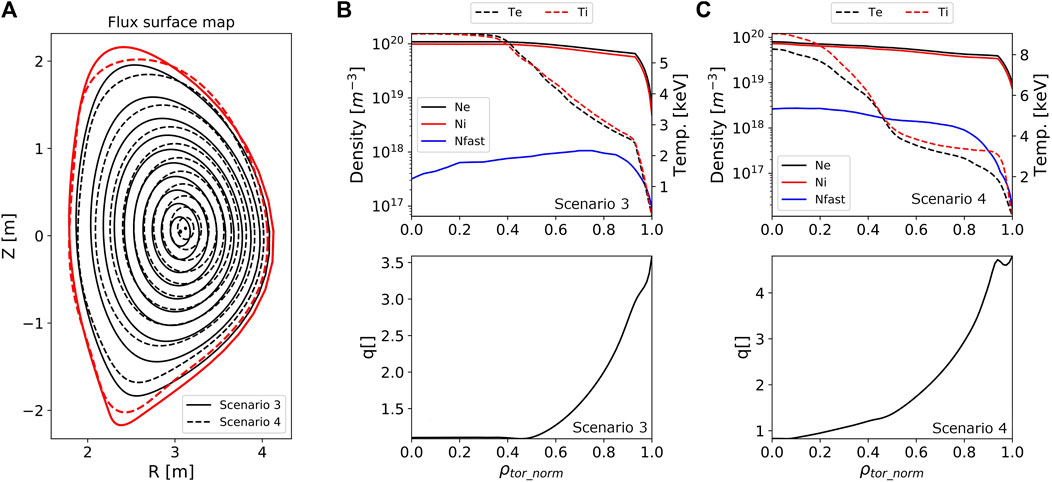FIGURE 1. Poloidal magnetic flux map with the plasma boundary highlighted in red for scenarios 3 and 4 (A), and electron/ion/energetic particle density and temperature and safety factor profile for operational scenario 3 (B) and hybrid scenario 4 (C).

In order to obtain the energetic particle distribution for each plasma scenario, the ASCOT code suite  was used. ASCOT is an orbit-following code, which uses the Monte Carlo approach to simulate the motion of charged particles in 3D magnetic fields and, together with appropriate collision operators, simulate the beam ionization (through the BBNBI code ), slowing down, and thermalization of the energetic ions toward a steady state. The simulations took into account the carbon impurity species in addition to the main deuterium ions from the plasma, and all energy components for positive-ion-based beams were included, i.e., E, E/2, and E/3. A thermalization “standard” end condition of 1.5 times the thermal energy was considered in the simulations. Separate runs were conducted for the positive-ion-based NBI (P-NBI) and negative-ion-based NBI (N-NBI) systems, powered at the foreseen nominal values (10 × 2 MW and 1 × 10 MW, respectively) to allow for an independent evaluation of the contribution of each NBI system to the Alfven eigenmode stability. P-NBI and N-NBI yield quite different deposition profiles, considering both the injection energies (85 keV for P-NBI and 500 keV for N-NBI) and geometries (predominantly perpendicular for P-NBI and only tangential for N-NBI). The different plasma densities in both scenarios under consideration also lead to evident differences, with the P-NBI deposition and slowing down clearly dominant closer to the plasma edge for scenario 3, as shown in Figure 2A (particle distribution integrated over velocity space). Since only two out of the 10 P-NBI units used have a tangential injection, the velocity pitch angle distribution is mostly symmetric in the parallel velocity, as shown in Figure 2C (particle distribution integrated over the spatial domain). The three different energy components are quite evident, showing a significant fraction of ions at their birth energies.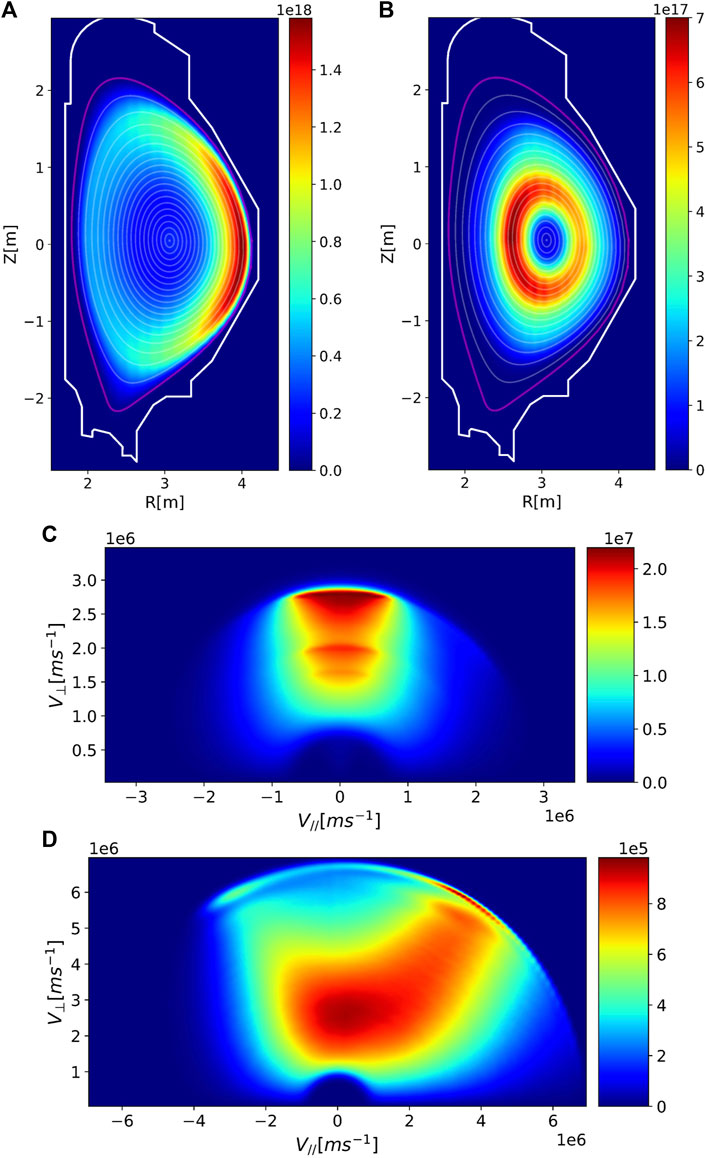FIGURE 2. Spatial distribution for the energetic particles from P-NBI (A) and N-NBI (B) beam sources calculated by the ASCOT code over scenario 3. Contour levels for the magnetic flux surfaces, plasma boundary, and first wall are also shown for convenience. Units are given in [m-3]. Also shown is the velocity distribution for the energetic particles from P-NBI (C) and N-NBI (D) beam sources calculated by the ASCOT code over scenario 3. Units are given in [s2m-2].

The N-NBI beams, on the other hand, penetrate deeper inside and yield roughly an annular distribution over the RZ plane (see Figure 2B) due to a significant fraction of a co-passing population (see Figure 2D). Figure 2C clearly shows that much like the P-NBI distribution, the distribution exhibits characteristic bump-on-tail features close to the birth beam energy of 500 keV, in the v//>0 region, due to tangential injection. From these observations, one can already anticipate the potential effect on the stability of the MHD shear Alfven waves associated with this scenario. Although the P-NBI distribution is primarily relevant for edge-localized modes, where the spatial gradient of the distribution function (proxy for the derivative with respect to Pphi) is largest in magnitude, the N-NBI distribution can potentially destabilize a larger pool of modes yet dominantly located from midradius outward where $∂FEP∂Pphi<0$ is anticipated. The maximum of the flux surface-averaged N-NBI distribution is, indeed, located at $s=ψN∼0.42$, where $ψN$ is the normalized poloidal magnetic flux. Averaged over velocity space, this yields a fast ion density of the order $6×1017m−3$, which is roughly 0.6% of the local bulk plasma density. The bump-on-tail features of the N-NBI distribution can potentially assist the drive of some modes unstable by co-passing particles through $∂FEP∂E>0$, something not as easily accessible to the P-NBI distribution except for deeply trapped particles.

For hybrid scenario 4, owing to the lower plasma density and similar electron/ion temperature from midradius outward, both P-NBI and N-NBI beams are expected to penetrate deeper inside the plasma volume, so a larger pool of modes, e.g., core localized modes, might be expected to interact with the energetic particle population. This is particularly relevant if we consider the ITB region ($ΦN∼0.3−0.5$ or $ψN∼0.4−0.6$) since fast ions are believed to play an important role in the stabilization of turbulence in this scenario , and fast ion redistribution resulting from destabilized Alfvén eigenmodes could potentially degrade ITB. Figure 3 shows the ASCOT distribution function over RZ and velocity spaces for the P-NBI (A, C) and N-NBI (B, D) settings, respectively. Although less evident, the singled-out bump on the tail of the N-NBI distribution close to the birth energy is again observed. As previously anticipated, the fast ions penetrate deeper into the plasma, yielding a non-negligible particle density on the axis.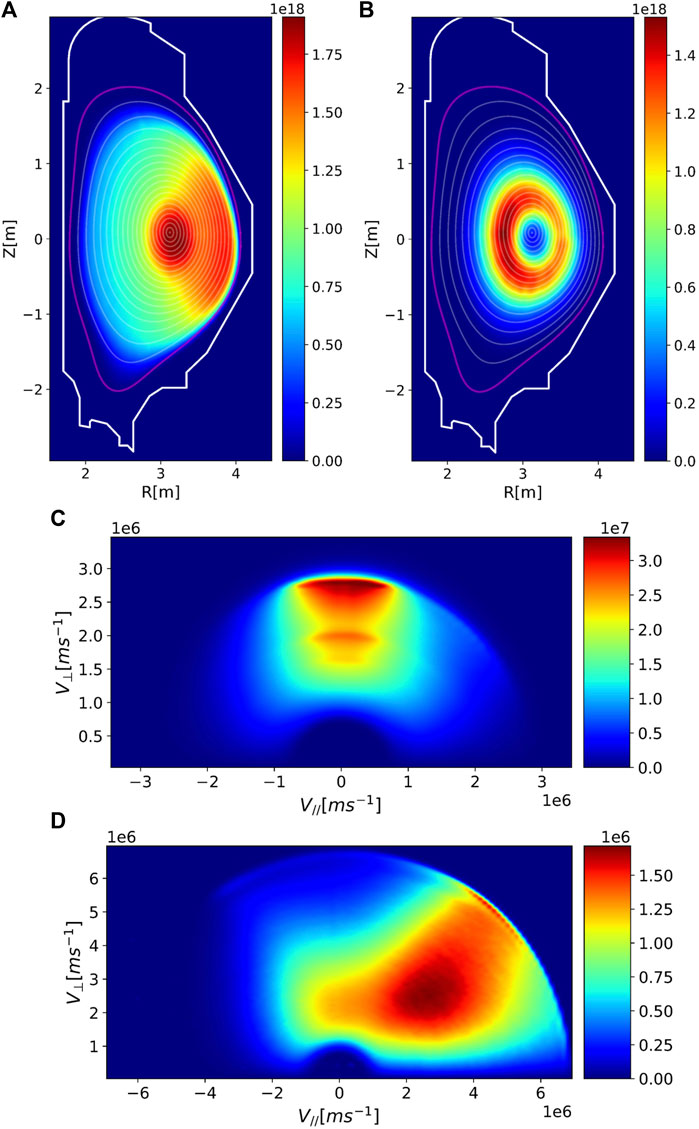FIGURE 3. Spatial distribution for the energetic particles from P-NBI (A) and N-NBI (B) beam sources calculated by the ASCOT code over scenario 4. Contour levels for the magnetic flux surfaces, plasma boundary, and first wall are also shown for convenience. Units are given in [m-3]. Also shown is the velocity distribution for the energetic particles from P-NBI (C) and N-NBI (D) beam sources calculated by the ASCOT code over scenario 4. Units are given in [s2m-2].

### 2.3 MHD and fast particle modeling framework

To investigate the linear interaction of the energetic beam particle populations with the possible pool of MHD Alfven eigenmodes in each scenario, a workflow  relying on the fixed-boundary high-resolution equilibrium code HELENA , the ideal incompressible MHD linear stability code MISHKA , and the hybrid MHD-drift kinetic code CASTOR-K [44, 45] is used. CASTOR-K uses a perturbative approach for the perturbed distribution function of the energetic ions associated with a given normal eigenmode of the MHD kernel (ideal/resistive) and, therefore, cannot be used to study energetic particle modes or any non-linear behavior of the mode amplitude and/or frequency. The perturbed Lagrangian used in the simulations assumes the MHD eigenmode to be incompressible; thus, this precludes the analysis of any modes where coupling with the sonic branch of compressible MHD occurs, e.g., BAEs. The model does, however, include finite particle orbit width effects and can, therefore, address all different types of orbits and orbit topological regions, e.g., trapped-passing boundary. Arbitrary distribution functions in the (Pphi,E,μ) constant of motion phase space (parametrized or from tabulated data) can be used in the model, which grants CASTOR-K the capability to address arbitrary fast ion sources such as fusion alphas or NBI/ICRH additional heating in any possible configuration, as well as thermal background ions. Since CASTOR-K code operates as an eigenvalue solver, it is much more adequate to analyze the damping rates of stable modes than initial value codes and sub-dominant modes at the same toroidal mode number and neighboring frequencies. It is also far less computationally intensive than any equivalent initial value code. The model only addresses kinetic effects from the thermal or energetic particle populations, which means that fluid damping due to conversion of the mode energy into strongly damped kinetic Alfvén waves (radiative damping) and collisional electron damping are not directly included in CASTOR-K but can be suitably calculated by a resistive MHD code (see Ref. 44). The identification of all AE solutions of the linearized MHD equations with MISHKA is carried out over a range of toroidal mode numbers (n = 1–25) and with normalized frequency ω/ωA within 0.01 and 2.7, where ωA is the Alfvén frequency on axis. The upper limit in frequency is a reasonable compromise, considering the maximum beam energy of 500 keV, the particle energy at the Alfven velocity (on axis) of EA∼136 and 150 keV for scenario 3 and hybrid scenario 4, respectively, and the ability to identify not only TAEs but also EAEs and NAEs. The upper limit in the toroidal mode number is somewhat optimistic, considering the inherent limitations to the drift-kinetic model over which the perturbative analysis of CASTOR-K takes place. To be strictly valid, the perpendicular wavevector of the perturbation k should satisfy $k⊥rL<1$, where $rL$ is the typical Larmor radius of the energetic particle. This translates into a maximum toroidal mode number $Nmax∼ s/qmode/rL/a$ with s as the normalized radial coordinate and the lower script “mode” as the radial location, where a particular mode has the energy density of the highest mode (hereafter referred to as “mode location”). Taking an indicative average particle energy E∼250keV, midradius mode location, and q∼1 yields Nmax∼15. As the mode locations and dominant particle resonances are largely unknown a priori, we consider this value of Nmax as a more realistic upper limit than the assumed n = 25, although, in the following sections, the results also cover modes up to n = 25. The pool of modes within the prescribed toroidal mode number and frequency range is then reduced by discarding all modes that cross the Alfvén continuum as the interaction of the modes with the continuum is considered to strongly damp the modes . In all CASTOR-K simulations, a 201 radial-by-256 poloidal plasma equilibrium grid was considered, and a 32-poloidal harmonic spectral resolution was considered when calculating the MHD eigenmodes. The minimum poloidal mode number depended on the toroidal mode number and on the safety factor q(s) on the axis to ensure that the most relevant resonance/gap modes, e.g., q=(m-1/2)/n up to s∼0.8, were covered for the modes of interest, i.e., below n∼15. CASTOR-K is executed independently over all this set using three different distribution functions: the thermal ion Maxwellian population, and the P-NBI and the N-NBI beam ion populations. When using the beam ion populations in CASTOR-K, these are first mapped appropriately to the (Pphi,E,μ) constant of motion space from the ASCOT output.

## 3 Results

### 3.1 Alfven eigenmode stability in scenario 3

In this scenario, a total of 213 AEs were identified, following the criteria detailed in Section 2.3, covering most of the frequency range and yielding modes localized from deep inside the core up to the plasma boundary (see Supplementary Figure S1 in Supplementary Material). When considering both the effect of the neutral beam energetic ions (PNBI + NNBI sources) and the thermal ions on the mode stability, it is observed, as shown in Figure 4, that all modes are found to be stable independent of their frequency (A) or location (B). Thermal ion Landau damping dominates and, whenever beam ions drive the modes unstable, it is roughly one order of magnitude lower, i.e., $γωA≲0.2%$ (see discussion in the following paragraph) for the N-NBI beams and $γωA≲0.02%$ for the P-NBI beams. Although the figure may suggest that there are fewer than 213 modes, there is a multiplicity of modes that have frequencies so similar that they appear to be overlapping. This is to be expected in low-shear regions of the plasma, as is the case of the plasma core, where multiple modes can be found within a single Alfven gap and with increasing radial wavenumber on the mode harmonics as the mode frequency approaches either lower or upper ends of a given Alfven gap .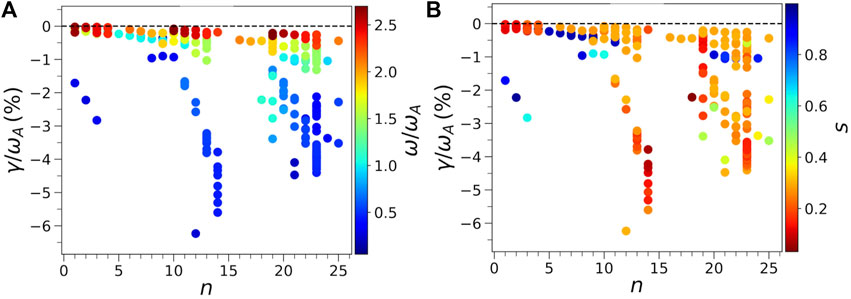FIGURE 4. Normalized growth rate of the Alfvén eigenmodes for scenario 3 for the toroidal mode numbers n = 1–25 when both thermal ion and NBI ion effects are considered. The color bar labels the modes, according to their normalized frequency (A) and radial location (B).

Although the combined effect of the thermal ions and energetic beam ions leads to stabilization of all identified Alfvén eigenmodes, it is important to investigate which particular modes are destabilized by the beam ions and identify the underlying features in the beam ion distribution function or wave–particle resonances that cause such destabilization. Since the P-NBI beam ions have a much less relevant impact on mode stability compared to N-NBI, here, we focus only on the driven/damped modes by the N-NBI beam ions. The overview results on the growth/damping rates stemming from N-NBI fast ions alone are shown in Figure 5, and one observes that, at most, one obtains a normalized growth rate $γωA∼0.2%$. In order to obtain the net drive when considering both thermal ion damping and potential NBI drive, we found that the threshold for fast ion density (nfast-crit) would need to be five times than expected in the scenario reference (nfast-ref), i.e., nfast-crit> 5nfast-ref, translating to 3% in the relative density of fast ions to thermal ions. From Figure 5, a first inspection confirms, as anticipated in Section 2.2, that the modes driven unstable are located at the s > 0.5 plasma domain where the fast ion distribution function has a negative radial gradient. However, this is a rather simplistic assessment since a) the magnetic flux of a magnetic surface is just a proxy for the canonical toroidal angular momentum Pphi and b) the drive for the mode excitation can equally arise from a positive gradient in energy of the distribution function if relevant wave–particle resonances are found at the bump-on-tail regions of phase space of the distribution (see Figure 2). Likewise, mode damping may well arise from local positive gradients of the distribution in Pphi, i.e., $∂FEP∂Pphi>0$, in addition to the usual $∂FEP∂E<0$ contribution.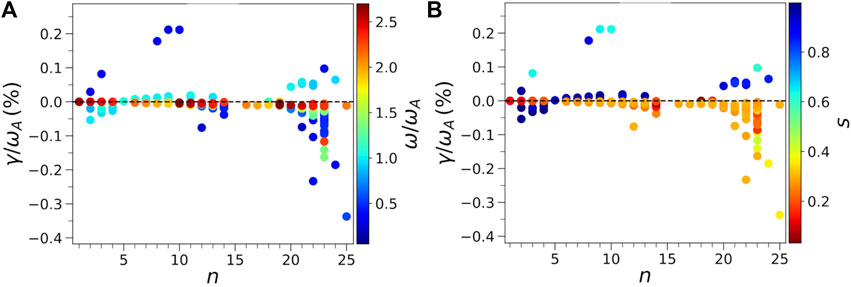FIGURE 5. Normalized growth rate of the Alfvén eigenmodes for scenario 3 considering only the N-NBI ions for the toroidal mode numbers n = 1–25. The color bar labels the modes according to their normalized frequency (A) and radial location (B).

As shown in the following sections for some representative modes, in the majority of the cases where the beam ions drive the modes unstable, wave–particle resonances located around the 500-keV birth energy of the beam ions play a relevant role in mode destabilization. In addition, the drive coming from $∂FEP∂E>0$ may be significant, as anticipated in bump-on-tail distributions (see Figure 2B when particle energy is close to maximum). Mode damping is also observed not to arise exclusively from $∂FEP∂E<0$, with wave–particle resonances crossing regions of phase space, where $∂FEP∂Pphi>0$, particularly relevant in the plasma core (see Figure 2B).

### 3.2 N = 9 destabilized TAE

A typical example of net mode drive by the energetic particle distribution of the AE spectra is evidenced by the n = 9 TAE mode with ω/ωA = 0.302. As shown as follows, it features most of the potentially different stability trends associated with the beam distribution from the N-NBI system. The mode is not radially localized but extends from midradius up to the plasma edge, owing to broad poloidal harmonic spectra as is usual when the magnetic shear is not small (see Figure 6A). For dominantly co-passing particles, the wave–particle resonances are located in the $∂FEP∂Pphi<0$ region of phase space, and thus, the EP distribution destabilizes the mode, with noticeable energy exchange around the birth energy of the injected neutral atoms (500 keV). This is shown in Figure 6B, where the distribution function and the dominant wave–particle resonance (magenta dashed curve) for the co-passing particle population (labeled as sigma = 1) for normalized magnetic moment $λ=μBE=0.4725$ are shown. As shown in Figure 6C, the highest energy transfer between particles and waves for the co-passing particle population (labeled as sigma = 1) occurs close to the bump-on-tail features at E∼500 keV, which clearly yields a destabilizing contribution stemming both from $∂FEP∂E>0$ and $∂FEP∂Pphi<0$ contributions. We note that in all the figures showing the distribution function over phase space (E,Pphi) for a given λ, the apparently coarse resolution of the domain is just a visual artifact arising from the symbol size used in the scatter plot and from the adaptive grid refinement used by the CASTOR-K code to calculate the total number of interacting particles, i.e., the grid need not have the same resolution in all phase space regions but rather be refined only where it matters the most.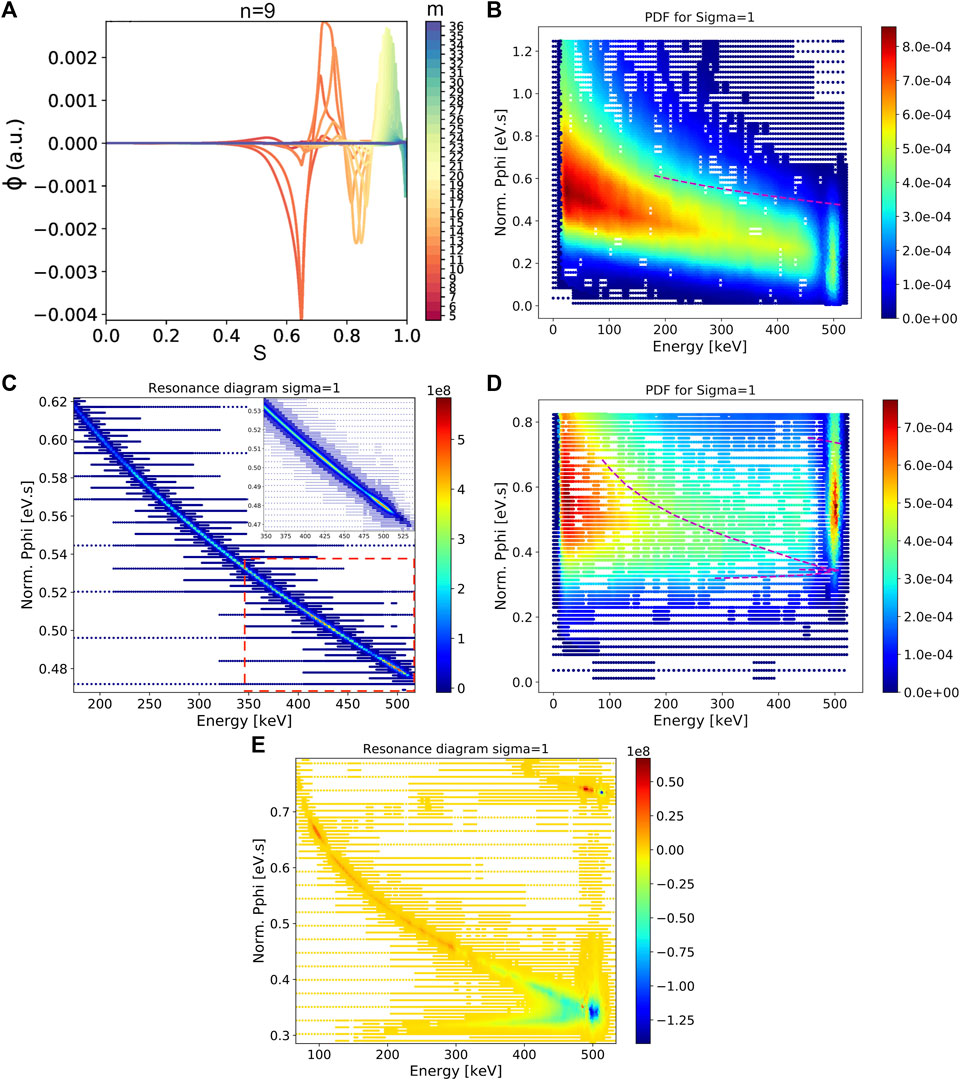FIGURE 6. Electrostatic potential eigenfunction for the n = 9 TAE mode with ω/ωA = 0.302 (A). The distribution function [s3m-6] for the N-NBI beam ion distribution modeled by ASCOT in phase space (E,Pphi) for co-passing particles at λ = 0.4725, as discretized by the mesh refinement algorithm of CASTOR-K, and the dominant wave–particle resonance (magenta dashed curve) is shown in (B). The resonance map in normalized CASTOR-K units in the energy exchange is also shown in (C). The wave–particle energy exchange is highest at the neutral beam ion birth energies (500 keV). The red dashed rectangle in the figure highlights the figure inset at the top right with detail over the highest wave–particle energy exchange. At λ = 0.7665, the distribution function [s3m-6] for the N-NBI beam ion distribution in phase space (E,Pphi) for co-passing particles is shown in (D). The wave–particle resonances dominating the interaction are highlighted by the four dashed magenta lines. The resonance map for co-passing particles (sigma = 1) evidencing the strong response close to the beam ion birth energy is shown in (E).

As λ is increased (increased relative weight of perpendicular velocity to the total particle velocity or energy), the wave–particle resonance gradually shifts to the lower end (in Pphi) of the distribution where a net stabilizing effect ensues, assisted by a positive gradient in Pphi. Similar to the case at λ = 0.4725, the bump-on-tail features contribute to the energy exchange (see Figures 6D, E for the resonance map, distribution function, and energy exchange at λ = 0.7665). The guiding center orbits exchanging the most energy with the mode are located within the innermost regions of the mode eigenfunction, coinciding with the highest EP density from N-NBI, as evidenced in Figure 7. It should be noted that as λ increases, the shape and magnitude of the distribution function also change, and the net result, once integrated over λ, turns out to be destabilizing.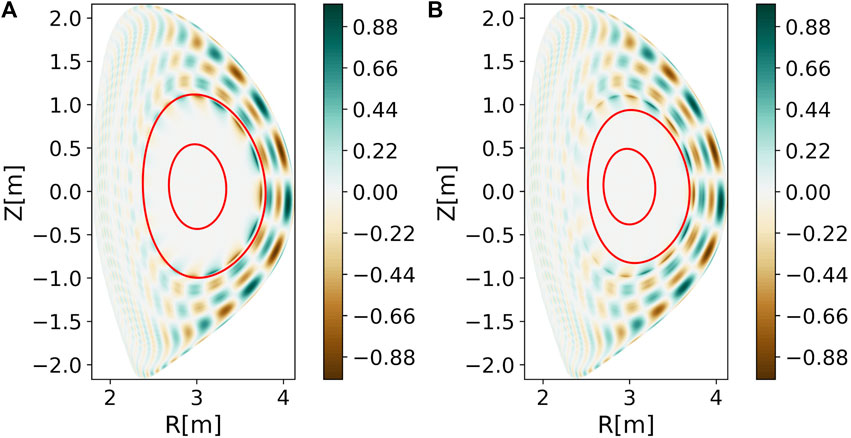FIGURE 7. Electrostatic potential eigenfunction for the n = 9 TAE mode with ω/ωA = 0.302 with an overlay of guiding center orbits (in red) at the highest (A) destabilizing (λ = 0.4725) and (B) stabilizing (λ = 0.7665) wave–particle energy exchange. For the given constant of motion, there are two orbits possible with the outer one being the co-passing orbit.

### 3.3 N = 12 stabilized TAE

The n = 12 mode considered is localized at the core, has a normalized frequency ω/ωA = 0.248, has a dominant m = 13 poloidal harmonic, and is the mode (n < 15) with the highest damping by N-NBI. The electrostatic potential is shown in Figure 8A. Owing to the quite central localization of the mode, wave–particle resonances above moderate energy ($E≳50keV$ are expected to be accessible primarily to counter-passing particles only. From the CASTOR-K calculations, stability is indeed dominated by counter-passing particles, with $∂FEP∂Pphi>0$ contributing significantly to the overall damping of the mode. Figure 8B shows the distribution of counter-passing particles (labeled as sigma = −1) for the normalized magnetic moment λ = 0.6825, where the absolute value of the differential in energy exchange (dWhot/dλ) has its maximum in the absolute value. The dominant resonance region (damping) is also highlighted in magenta and occurs at Pphi∼0.28 eVs and E∼90keV, corresponding to the vA/3 resonance. When looking for the highest energy exchange over phase space, at the dominant wave–particle resonance, one easily confirms that the positive gradient in Pphi term of Eq. 2 (Figure 8D) contributes much higher to mode damping than the negative gradient in energy term (Figure 8C). A cross section of the electrostatic potential of this n = 12 TAE over the (R,Z) space is shown in Figure 8E, together with the two possible orbits for the constant of motion (Pphi = 0.28 eVs, E = 90 keV, λ = 0.6825), where damping from the energy gradient is largest. As shown in Figure 8B, these represent a small fraction of phase space for the energetic particle distribution, and thus, the overall damping is unsurprisingly smaller compared to the N-NBI drive of the n = 9 mode presented in Section 3.2. At lower values of λ, a similar pattern of wave–particle resonances is observed, although at slightly lower energy and toroidal angular momentum and with vA/3 still acting as single/dominant resonance.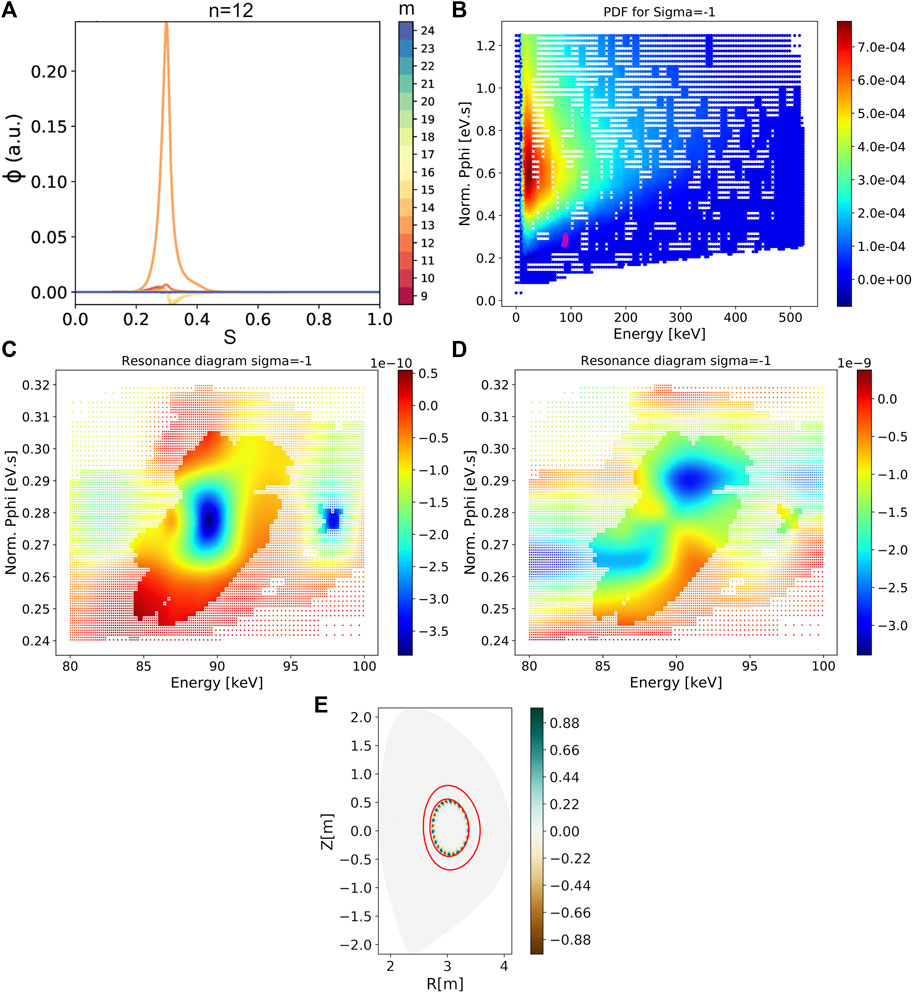FIGURE 8. Electrostatic potential eigenfunction for the n = 12 TAE mode with ω/ωA = 0.248 (A). Distribution function [s3m-6] for the N-NBI beam ion distribution modeled by ASCOT in phase space (E,Pphi) for counter-passing particles at λ = 0.6825, as discretized by the mesh refinement algorithm of CASTOR-K (B). The wave–particle resonance dominating the interaction of the mode is highlighted with the magenta footprint at E∼90 keV and Pphi∼0.28. The energy gradient term $ω∂FEP∂E$ (C) and Pphi gradient term $−n∂FEP∂Pphi$ (D) of growth rate (Eq. 2) at the main wave–particle resonance are also shown. The electrostatic potential eigenfunction of the mode with an overlay of guiding center orbits (in red) at the highest stabilizing wave–particle energy exchange from the distribution energy gradient is shown in (E). For the given constants of motion, the outer orbit is the co-passing orbit.

### 3.4 Sensitivity scan on the safety factor

To model a possible uncertainty in the q-profile, a variation of ±1% was considered on the total plasma current around the reference value. In the ensuing analysis, only the plasma equilibrium is recalculated, i.e., the same thermal density/temperature profiles and energetic particle distribution functions as the reference scenario were considered. Although these slightly different scenarios are, thus, not fully self-consistent, the emphasis of the analysis is on the qualitative impact on the mode spectra and possible impact of the drive/damping nature of the interaction between the energetic distribution functions and the modes. The calculated equilibria for the three scenarios have, as expected, negligible differences in the flux surfaces mapping in (R,Z), considering the small variation in plasma current and/or in the equilibrium pressure, i.e., no change in the Shafranov shift is observed and the relative change in the q-profile is almost hardly noticeable (see Figure 9).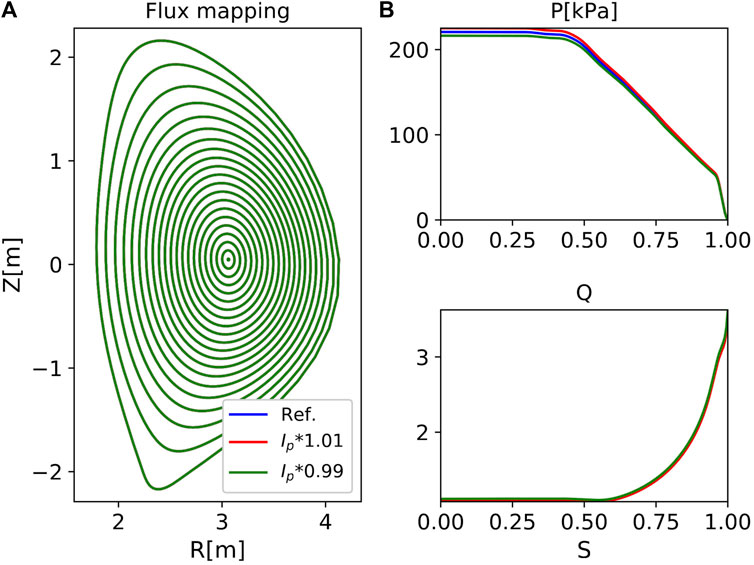FIGURE 9. Flux surface map (A), plasma pressure, and safety factor profile (B) for reference (Ref.) scenario 3 and for neighboring equilibria with the plasma current scaled up/down by 1%.

Given the shape of the q-profile, which exhibits very low shear in the core, and the fact that Alfvén eigenmode spectra are highly dependent on the local q-profile features, it is anticipated that the effect of the small changes in plasma current on the eigenmode spectrum is most prominent for modes localized at the plasma core. This is confirmed when the total spectra of modes that do not cross the Alfvén continuum are plotted, as shown in Figure 10. Although modes located outside the low-magnetic shear region have very small variations in frequency, core modes show significant variations in frequency and, in some cases, are eliminated from the analysis since, albeit small, the change in the safety factor can cause the frequency of some modes to touch neighboring branches of the modified Alfvén continuum.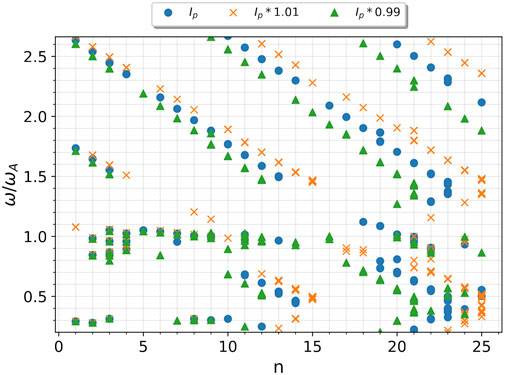FIGURE 10. AE spectra for the reference and ±1% variation in toroidal plasma current scenarios. Only modes not crossing the Alfvén continuum are retained. The upper/lower shift in mode frequencies impacts only the core localized modes.

Regarding MHD stability, there is little change overall since thermal ion landau damping still dominates the wave–particle interaction, and both 1% increase and decrease in plasma current still yield stabilized modes (see Supplementary Figure S2). Similar to the reference equilibrium, the variants of 1% in plasma current also evidence mode destabilization by the N-NBI beam ions. It was verified in the simulation results that the unstable modes typically have mode numbers n = 3,7–10, their poloidal harmonic content is localized mostly at s > 0.6, and the mode drive comes primarily from the co-passing population with normalized magnetic moment λ = [0,0.65]. In the (Pphi,E) space, the drive arises once more from wave–particle resonances at the downslope region of the distribution function, i.e., where $∂FEP∂Pphi<0$, extending up to the birth energy of the beam ions. The bump-on-tail features of the distribution assist in the driving/damping of the mode whether particle energy is slightly smaller/higher than the birth energy, similar to the results already obtained for the reference scenario.

### 3.5 Alfven eigenmode stability in scenario 4

In this scenario, a total of 358 AEs were identified, following the criteria detailed in Section 2.3. Similar to the full Ip inductive scenario 3, very few modes (here, only EAEs with a frequency of the order of the Alfvén frequency) were found in the region where the ITB is located, i.e., s = $ψN∼0.4−0.6$, as shown in Figure 11B. One notices that there is a reasonable number of modes localized very close to the axis (see Figure 11B). Such modes are, in some cases, ill-resolved radially and not considered in the MHD stability analysis. The scarcity of modes within the ITB region is encouraging as the absence of Alfvén eigenmodes in that region eliminates the possibility of fast ion transport induced by a resonant interaction with these modes. However, it also raises the question of why no modes are present within ITB. Is the bulk plasma pressure gradient so large that no shear Alfvén wave solutions are permitted in the linearized MHD equations , or do some of the existing solutions cross the Alfvén continuum and are, thus, excluded from the analysis?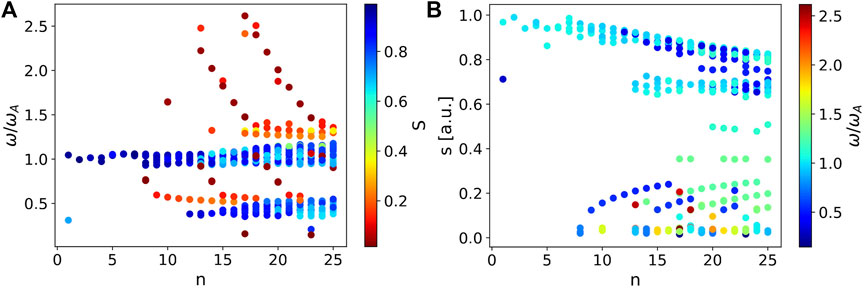FIGURE 11. Alfvén eigenmode spectra for scenario 4 for the toroidal mode numbers n = 1–25. The y-axis indicates the mode frequency normalized to the Alfvén frequency (A) or the mode location (B), and the color bar labels the modes according to their radial location and normalized frequency, respectively.

In order to explore the former hypothesis, the ideal MHD spectra were re-calculated using two scaled variants of the reference equilibrium where the plasma beta (pressure profile) was scaled down to 70% and 50%, respectively. The analysis evidenced that there is no increase in the number of modes in the radial range of ITB (which do not eventually cross the continuum) when decreasing plasma beta (see Figure 12 showing only modes with a normalized frequency below 1). Moreover, all modes located at s > 0.6 with a frequency close to ω/ωA = 1, corresponding to the EAEs shown in Figure 11, are still observed. One should note that the imposed beta scaling of the equilibria does impact the safety factor q(s) profile even though the total plasma current only shows a variation below 0.1%. This causes slight variations in the mode locations, similar to the plasma current scan performed for scenario 3 (see Figure 10).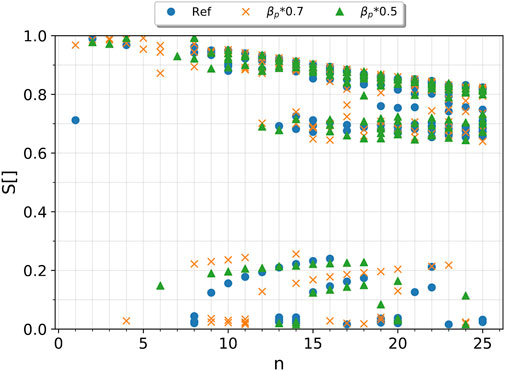FIGURE 12. Alfvén eigenmode spectra for the reference (full circles), 70% (crosses), and 50% (triangles) plasma beta for scenario 4, as ordered by the mode location for mode frequencies below ω/ωA = 1.

In order to confirm that the local absence of TAE modes is a continuum effect, it is instructive to plot the Alfvén continuum for a representative subset of the toroidal mode spectra, e.g., n < 10, to avoid cluttering the plot. As shown in Figure 13, since magnetic shear is not small and considering the equilibrium mass density and q-profile, most TAE gaps in the range $s≳0.25$ are relatively close to each other and do not have gap extrema aligned in frequency. This implies that gap modes either touch the continuum at inner radii, e.g., 0.25 < s < 0.6 gap modes, or touch the continuum at s∼0.9. Two representative examples (n = 5 and n = 7) are shown in Figure 14. When n = 5 with ω/ωA = 0.525 (Figure 14A), although the frequency matching the Alfvén continuum occurs only near the edge, it is unavoidable that the relevant (“resonant”) poloidal harmonics of the eigenfunction exhibit sharp local variations over radius and are, thus, discarded. When n = 7 with ω/ωA = 0.401 (see Figure 14B), the continuum crossing occurs at a radial location where the poloidal components of the eigenfunction still hold significant energy, the sharp radial variations in the eigenfunction are much more noticeable, and thus, the mode is trivially discarded. One notes that there are other eigenfunction solutions for n = 7 (extensible to other toroidal mode numbers) which feature dominant m=(n-1),n poloidal harmonics, which resonate strongly with a continuum close to the TAE gap centered at s∼0.2–0.3. In such cases, these harmonics overshadow the remaining poloidal harmonics (barely noticeable in the eigenfunction radial profile). Figure 13 shows that as the toroidal mode number increases, at 0.25 < s < 0.6, it only allows for sufficiently localized (high-n) of odd parity modes at the top of the gap ω/ωA ∼[1,1.3], in agreement with the results shown in Figure 11B.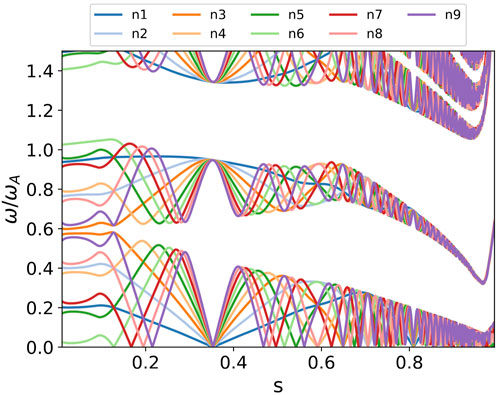FIGURE 13. Alfvén continuum for the reference scenario 4 equilibrium and toroidal mode number below n = 10. Core TAE modes are only allowed below s ≈ 0.25.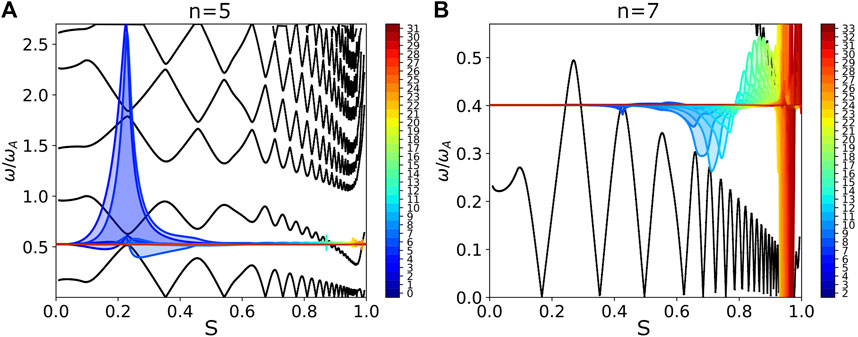FIGURE 14. Electrostatic potential eigenfunction and continuum spectrum for n = 5 with ω/ωA = 0.525 (A) and n = 7 with ω/ωA = 0.401 (B) modes, which cross the Alfvén continuum to the right of the ITB region (s∼[0.4,0.6]. The mode frequency closely matches the lower limit of the TAE gap in the core (n = 5) and in the vicinity of s∼0.4 (n = 7).

When the contribution from N-NBI, P-NBI, and thermal ions to the mode stability is calculated, similar to scenario 3, all modes are observed to be stable (Figure 15A). However, in scenario 4, there is a more sizeable number of modes which are driven unstable by N-NBI, as shown in Figure 15B, showing the stability overview results including only the contribution from N-NBI fast ions. Such modes are primarily in the TAE and EAE frequency ranges (not shown) and are all located in the outer regions (s > 0.6) of the plasma. Although thermal ion landau damping is smaller than at the core, it is enough to overcome the beam drive.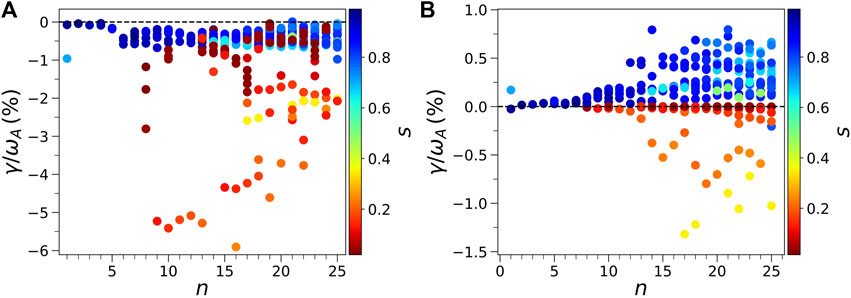FIGURE 15. Normalized growth rate of the Alfvén eigenmodes for scenario 4 with a neutral beam and thermal ion contributions (A) and with the N-NBI contribution alone (B) for the toroidal mode number n = 1–25. The color bar labels the modes according to their radial location.

## 4 Discussion

In this work, a detailed review of the expected energetic particle-driven MHD shear Alfvén instability phenomenology of variants of the two main operational scenarios for the JT-60SA tokamak is presented. The energetic particle populations arising from both normal and tangential NBI beam units in the device are shown to have just a moderate effect on the MHD stability, most notably for the N-NBI (tangential) 500-keV beams. Except for the normal injection beam contribution from P-NBI in scenario 4, beam power deposition in the plasma is peaked off-axis. In scenario 3, this translates to the most notable examples of driven modes by the N-NBI beam ions to be located from mid-radius outward. The drive $γωA≲0.2%$ from primarily co-passing beam ions is shown to arise from wave–particle resonances in the (E,Pphi) phase space where the bump-on-tail feature in the beam ion population plays a dominant, although not exclusive, role. The drive is, however, low compared to the ion Landau damping, which is shown to be significant for an ion temperature $Ti≳3keV$ in the region where the driven mode amplitude is most noteworthy.

Hybrid scenario 4 was also investigated, focusing, in particular, on the stability of AEs located in the vicinity of the ion energy ITB which, coincidentally, matches the region of the highest density of beam ions from the N-NBI system. When analyzing the mode spectra for a large range of toroidal mode numbers, it was shown that very few modes are found in the vicinity of the ITB region, which is an encouraging result since it can potentially mitigate fast ion transport and, thus, aid the sustainment of the transport barrier. Although some of the modes found are driven unstable by the N-NBI beams, it is clearly insufficient to overcome the stabilizing contribution of the thermal ion Landau damping. When extending the analysis to the entire spectrum of modes, the entire set is found to be stable, again with ion Landau damping dominating. The underlying causes for the scarcity of modes at the ITB region were investigated. The MHD mode spectra of rescaled background plasma pressure variants of the equilibrium were calculated and revealed that the absence of modes is not related to the magnitude of the plasma pressure gradient. Rather, it is a consequence of an unfavorable Alfvén continuum, which blocks many of the TAE and EAE gaps except at the deep core and edge regions of the plasma.

The analysis presented makes some relevant assumptions which, in future work, should be relaxed or reconsidered. First, the scenario variants are all analyzed in the plasma current flat top steady state. During current ramp-up assisted by NBI, it is conjectured that EP-driven modes might actually be driven unstable  and, thus, interfere with the establishment of the steady-state scenario, e.g., prevent the ITB formation itself in scenario 4 or achieving the nominal fast ion pressure expected for scenario 3, and thus, modify the final steady-state total plasma pressure, current density, and, ultimately, the q-profile. Analysis of the time-dependent equilibria and fast ion deposition profiles from self-consistent integrated modeling simulations on the scenarios’ current ramp-up would, therefore, be essential to predict/validate the predicted steady-state scenarios. Second, the stability analysis was performed using ideal incompressible MHD models, and only modes with frequency not intersecting the Alfven continuum and, thus, not exhibiting the characteristic logarithmic singularity in some poloidal harmonics of the eigenfunction were retained for the stability analysis with CASTOR-K. In the analysis of scenario 4, this resulted in the exclusion of all TAE frequency range modes, which could lie within the ITB region and slightly inward at the core. This exclusion particularly impacted low toroidal mode numbers since, given the moderate plasma magnetic shear, the eigenfunction of low-n modes easily couples many poloidal harmonics, and eventually, one can resonate with the Alfven continuum. Future work should investigate the impact of using resistive incompressible MHD models, e.g., CASTOR , and estimate the actual continuum damping rate and other possible effects, e.g., collisional and radiative damping, in order to estimate the total damping on the modes.

## Data availability statement

Numerical data that support the outcome of this study are available from the corresponding author upon reasonable request.

## Author contributions

RC: conceptualization, data curation, formal analysis, investigation, methodology, software, supervision, visualization, and writing–original draft and review and editing. PV, MV, and KS: data curation, investigation, software, and writing–review and editing. PR: methodology, software, supervision, validation, and writing–review and editing. ET: methodology, software, and writing–review and editing. JG: data curation, investigation, software, supervision, and writing–review and editing. DB: formal analysis, methodology, software, supervision, validation, and writing–review and editing. FN and RC: writing–review and editing. JF: data curation, methodology, and writing–review and editing. AF: methodology, software, and writing–review and editing.

## Funding

The author(s) declare financial support was received for the research, authorship, and/or publication of this article. IST activities also received financial support from “Fundação para a Ciência e Tecnologia” through projects UIDB/50010/2020 and UIDP/50010/2020. This work was partially carried out using EUROfusion High Performance Computer Marconi-Fusion through EUROfusion funding.

## Acknowledgments

This work was carried out within the framework of the EUROfusion Consortium, funded by the European Union via the Euratom Research and Training Program (Grant Agreement No. 101052200—EUROfusion). Views and opinions expressed are, however, those of the author(s) only and do not necessarily reflect those of the European Union or the European Commission. Neither the European Union nor the European Commission can be held responsible for them. The corresponding author also acknowledges useful discussions with ASCOT team developers Seppo Sipilä and Antti Snicker.

## Conflict of interest

The authors declare that the research was conducted in the absence of any commercial or financial relationships that could be construed as a potential conflict of interest.

## Publisher’s note

All claims expressed in this article are solely those of the authors and do not necessarily represent those of their affiliated organizations, or those of the publisher, the editors, and the reviewers. Any product that may be evaluated in this article, or claim that may be made by its manufacturer, is not guaranteed or endorsed by the publisher.

## References